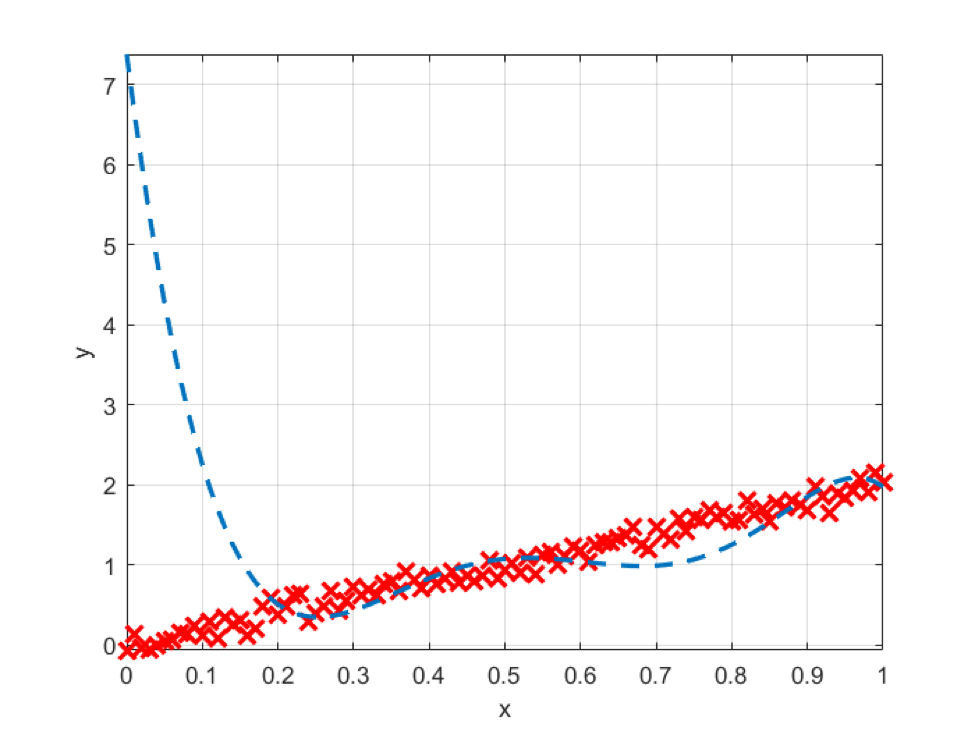top of page
Search
•Guy Regev

# understanding overfittingOverfitting is a frequently used term in the data science community; yet the most common explanation or “definition” that I hear regarding what Overfitting is usually goes something like this: “when the model learns the noise” or “when the model accuracy on the training set is very high but very low on the test set”.

First, let’s focus on the term “fitting”. Fitting in mathematics (i.e., curve fitting) is the process of finding a mathematical function that best describes (fits) a plurality of data points. Best in what sense? That’s outside the scope of this blog post, but suffice it to say that we are trying to minimize some error in order to achieve the most precise fit.

Hence, Overfitting is the term that is used to describe the application of a function that “perfectly” fits the data. Perfect in what sense? ~Zero error (or ~100% accuracy). The quotes are used to denote the fact that although the error is close to zero and the accuracy is apparently sky high, and the function is “too complex” for the data and hence the overfit. Let me illustrate that.

Consider an underlying linear model, as illustrated in Fig.1 below.Fig.1 - The data - The underlying model is linear. (y = 2x).

Further, suppose that we have only observed five points of this process (as depicted in Fig.2 below).Fig.2 - We observe only 5 points of the above process.

Now, let’s assume that we train a polynomial model of order 5. Then we’ll get a perfect fit, as seen in Fig.3.

But since we know that the underlying model is a polynomial of order 1 (linear), we know that the trained model is far from representing the data accurately. Fig. 4 below shows exactly that.Fig.3. - Data is overfitted by a polynomial of order 5.Fig.4 - The “overfitted” model does not represent the data. We know that the best model here is linear.

That is the problem of overfitting. We see that in a lot in deep learning applications because we generally have a lot of more trained parameters than training data points.

We can consider some ways to overcome the problem of overfitting:

1. Shrink the model size - There’s a significant body of work on a topic called “model order selection”. I refer the readers to an excellent paper on this topic.

2. Regularization - Not a new subject of research, and certainly not unique to machine learning. (e.g. Tikhonov regularization)

3. Get more data. (synthetic, augmentation, etc.)